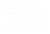This page contains content no longer in the game. This feature was removed and exists only in previous versions of 7 Days to Die.

## Overview

The Pure-Durability or Pure-Dur describes how many times a regular Zombie like the Infected Survivor may punch a block before breaking it.

## Calculation

```  Pure-Dur = RoundUp(Hardness ÷ 0.075)
```

## Examples

80 ÷ 0.075 = 1066.667
RoundUp(1066.667) = 1067
The Pure-Dur of a Steel Blocks is 1,067.
16 ÷ 0.075 = 213.333
RoundUp(213.333) = 213
The Pure-Dur of Reinforced Concrete Blocks is 213.
12 ÷ 0.075 = 160.0
RoundUp(160.0) = 160
The Pure-Dur of Concrete Blocks is 160.
12 ÷ 0.075 = 160.0
RoundUp(160.0) = 160
The Pure-Dur of Cobblestone Blocks is 160.
8 ÷ 0.075 = 106.667
RoundUp(106.667) = 107
The Pure-Dur of a Wood Frames is 107.
4 ÷ 0.075 = 53.333
RoundUp(53.333) = 53
The Pure-Dur of Reinforced Wood Blocks is 53.
4 ÷ 0.075 = 53.333
RoundUp(53.333) = 53
The Pure-Dur of Wood Blocks is 53.
3.5 ÷ 0.075 = 46.667
RoundUp(46.667) = 47
The Pure-Dur of Flagstone Blocks is 47.